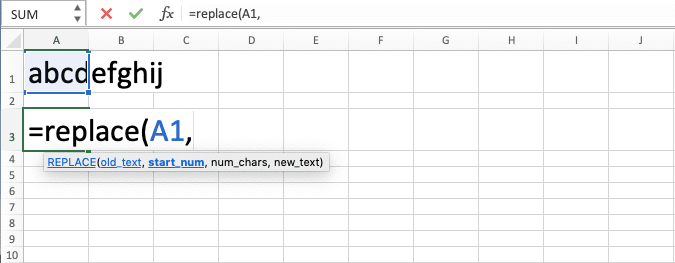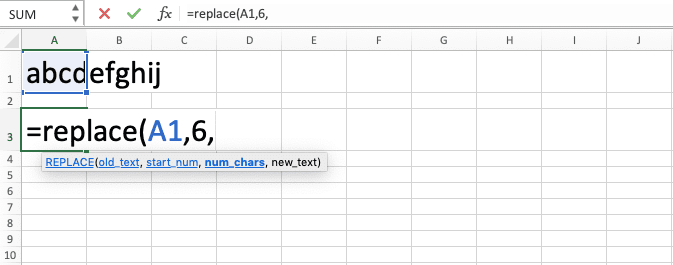How to Use the REPLACE Function in Excel: Usabilities, Examples, and Writing Steps - Compute Expert

# How to Use the REPLACE Function in Excel: Usabilities, Examples, and Writing Steps

Home >> Excel Tutorials from Compute Expert >> Excel Formulas List >> How to Use the REPLACE Function in Excel: Usabilities, Examples, and Writing Steps

In this tutorial, you will learn how to use the REPLACE function in excel completely.

When working with our data in excel, we may sometimes want to replace its part with something else. One of the methods you can use to do it is by utilizing this REPLACE function.

Want to know more about REPLACE and master the way you can use it properly in excel? Read this tutorial until its last part!

Disclaimer: This post may contain affiliate links from which we earn commission from qualifying purchases/actions at no additional cost for you. Learn more

## What is the REPLACE Function in Excel?

REPLACE is an excel function that helps you to replace a part of your data with something that you want. REPLACE will do its replacement process based on the data part position and its number of characters.

## REPLACE Usability

You can use REPLACE to replace a part of your data based on its position and number of characters.

## REPLACE Result

The REPLACE result is data which part you have replaced with something else.

## Excel Version from Which We Can Start Using REPLACE

We can use REPLACE in excel since excel 2003.

## The Way to Write It and Its Inputs

Here is the general writing form of REPLACE in excel.

= REPLACE ( data , position_of_data_part_to_replace , number_of_characters_of_the_data_part_to_replace , data_part_replacement )

And here is a bit explanation of the inputs you need to give when you write REPLACE in excel.
• data = the data which part you want to replace with something else you want
• position_of_data_part_to_replace = the number which represents the position of the data part you want to replace in the data
• number_of_characters_of_the_data_part_to_replace = the number which represents the number of characters of the data part you want to replace
• data_part_replacement = the replacement for the data part you want to replace in the data

## Example of Its Usage and Result

Here is an implementation example of REPLACE in excel.As you can see in the example, we can replace a part of our data easily with REPLACE. Just input the data, the data part position, number of characters, and its replacement to REPLACE. Press enter and you will get new data that already has the replacement for your data part in place.

When you input the data part position and number of characters to REPLACE, don’t forget to count things like spaces too.

You can also use REPLACE to remove a data part as you can see in the fifth row of the example. Just input a blank cell or empty quotes ( “” ) in your REPLACE replacement input part to do this.

## Using REPLACE Function in Excel

After we have discussed the REPLACE writing form, inputs, and implementation example, now let’s discuss its writing steps. We give an example screenshot for each writing step here so you can understand the step much easier.

1. Type an equal sign ( = ) in the cell where you want to put your REPLACE result2. Type REPLACE (can be with large and small letters) and an open bracket sign after =3. Input the data which part you want to change with something else. Then, type a comma sign ( , )4. Input the number that represents the position of the data part you want to replace in your data. Then, type a comma sign5. Input the number the number of characters of the data part you want to replace. Then, type a comma sign6. Input the replacement you want for the data part you want to replace7. Type a close bracket sign8. Press Enter
9. Done!## REPLACE vs SUBSTITUTE

There is another formula in excel you can use to replace a part of your data with something else. That formula name is SUBSTITUTE.

If that is so, then what is the difference between this SUBSTITUTE formula and REPLACE? Which is the best formula we should use for our data part replacement process in excel?

Well, first of all, let’s take a look at how we write SUBSTITUTE in excel.

= SUBSTITUTE ( data , data_part_to_replace , data_part_replacement , [ data_part_instance_to_replace ] )

As you can see there, there is a difference in the things we input to SUBSTITUTE in comparison to REPLACE.

If you use SUBSTITUTE, then you need to input the data part you want to replace directly. You can also input the instance of the data part you want to replace in your data. If you don’t input that, then SUBSTITUTE will replace all the instances of the data part in your data.

Thus, with that explanation, you may have already known the key difference between REPLACE and SUBSTITUTE. REPLACE replaces our data part based on its position and number of characters. Meanwhile, SUBSTITUTE replaces our data part based on its instances.

Therefore, if you want to determine the position and the number of characters to replace in your data, use REPLACE. However, if you want to determine the data part instance to replace (or if you want to replace all instances of the data part in your data), use SUBSTITUTE instead!

Here is an implementation example of SUBSTITUTE to make the formula concept clearer for you.As you can see there, we can use SUBSTITUTE to replace a part of our data with something else too. However, we base our data part replacement process on instances if we use SUBSTITUTE. We also need to input the data part we want to replace directly to the formula.

Whenever you need to replace your data part in excel, choose REPLACE/SUBSTITUTE based on the best one for your situation! If you have understood the differences between the two based on our discussion, you should be able to do that correctly.

## Exercise

After you have learned how to use REPLACE in excel completely, now let’s sharpen your understanding by doing the exercise below!

### Instructions

Use REPLACE to do all the instructions below!
1. Change the three characters before the first dash in the left code with “XYZ”!
2. Change one character after the first dash and before the second dash in the left code with “5”!
3. Change the eight characters after the second dash in the left code with “08092020”!

If you want to replace your data part in a non-case sensitive manner, you should use REPLACE rather than SUBSTITUTE. SUBSTITUTE is case-sensitive when it runs its data part replacement process.

Related tutorials you should learn from:

Get updated excel info from Compute Expert by registering your email. It's free!